If you have to debug a DSP algorithm, there are a couple of tools available to you. We’ve already discussed grabbing data samples and calculating histograms. We’ve also discussed taking FFTs of your data. But what if you want to estimate the spectral content of a signal within your FPGA on a platform that doesn’t have the resources to accomplish a full FFT?Certainly an FFT is the ideal operation to estimate spectral content: window a segment of an incoming signal, perform an FFT on that segment and then magnitude square each of the output samples for visual effect. Is the result too “noisy”? If so, you can average several FFTs together so that you can “see” the spectral shape of what’s going on in your environment. It’s just that all this comes at a cost. In my own pipelined FFT implementation, each stage requires between one and six multiplies together with enough block RAM to hold an FFT. At `log_2(N)` stages per FFT, this resource requirement can start to add up.

On the other hand, what if I told you that you could get (roughly) the same spectrum estimate for only the cost of a single DSP element, about two FFT’s worth of block RAM and some LUTs to control the whole logic? Well, that and a nearby CPU?

How about if I do one better, and say that the algorithm is easier to debug than an FFT? (FFT internals are notoriously painful to debug.)

There’s no real trick up my sleeve here, nothing more than plain old good engineering practice: if resources are tight in one location, such as in an iCE40 FPGA SDR application, you just move the resources you need to another location where they aren’t nearly as tight.

Let’s take a peek today at how we can move the spectral estimation problem from the FPGA to a nearby CPU without sacrificing (much) of the FFT’s capability.

## The key: Wiener-Khinchin Theorem

The key trick to this whole exchange is the Wiener-Khinchin theorem. The Wiener-Khinchin theorem states that the Fourier transform of the autocorrelation function is equivalent to the Power Spectral Density function. Using this principle, we can estimate the autocorrelation of a signal and then later take an FFT of the autocorrelation to get our Power Spectral Density estimate.

Let me back up, though, and start out closer to the beginning. Imagine you are in a lab and you have a signal you want to analyze. Perhaps you want to verify the shape of a low-noise amplifier, or find out whether or not there’s any interference from your digital electronics into some analog band, whether the signal you are transmitting from across the room made it into the receiver, or for that matter why your algorithm isn’t working no matter what it is. What would you do? You would place your signal into a spectrum analyzer and examine it’s content by frequency on the screen.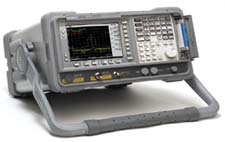The modern day digital spectrum analyzer will take a snapshot of that signal, possibly window the snapshot somehow, and then plot the Fourier transform of the result on the front panel. Mathematically, this operation looks somewhat like,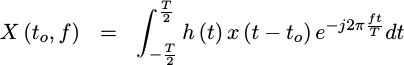where `x(t)` is the received waveform you wish to examine, `h(t)` is any potential window function, `T` is the length of the observed window, `t_o` is the middle of the snapshot window and `1/T` is the spectral resolution you have requested on the front panel. The device then plots this `|X(t_o, f)|^2` value against an `f/T` horizontal axis.

At this point, I would typically look at the screen and convince myself the spectral estimate is too “noisy” to do anything with, and so I’ll up the number of averages. Let’s try `N` averages.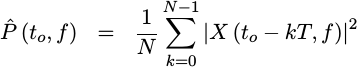Here, the plot settles out quite nicely and you have a reasonably good estimate of what the spectrum of your incoming signal looks like.

What we’ve just done is to estimate the Power Spectral Density of our incoming waveform.

The Power Spectral Density is defined as the expected value of the FFT of your signal, magnitude squared, as the size of the FFT grows without bound.For those who aren’t familiar with probability and statistics, an expected value is just an average. For those who are, please forgive my heresy, I just misspoke. An expected value has a very specific mathematical definition that depends upon the probability density function of the underlying random value. Specifically, the expected value of something, noted below as `E{x}` for the expected value of `x`, is defined as the integral of that something times the power spectral density of the random variable, shown below as `f_x(u)`, across its range, shown below by the infinite limits of the integral.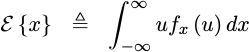Taking “averages” only estimates the true mathematical and underlying expected value–a difference which will come back to haunt us when we try to test today’s solution.

If you are willing to bear with me for a little math, let’s see if we can simplify this expression for the PSD into something more useful. The first step to doing so would be to expand out the conjugate squaring operation that takes the absolute value squared of the FFT within. Unfortunately, this will leave us with a nasty double integral.At this point, we can move the expected value inside the integrals and replace the `x^{*}(t_1)x(t_2)` expression with the autocorrelation function of our signal. This will require a change of variables in a moment.

For now, let’s define the autocorrelation function of a signal as the expected value of our signal multiplied by itself at some offset.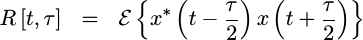You may note that I’ve chosen to place `t` between the two points that are multiplied together. This follows from Gardner’s work on cyclostationary signal processing, although we’ll adjust things back to the more familiar form next.

In particular, if our signal is wide sense stationary (WSS), this function will be independent of time. That means we can remove the time dependence of the autocorrelation and just represent it as a function of time difference, or `tau` which is also called the “lag”.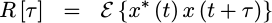(As a side note, I’ll just point out that no observed signal has truly been WSS, but the approximation tends to simplify the math. As I mentioned above, I personally find a lot of meaning in cyclostationary signals, where `R[t,tau]` isn’t independent of `t` but is rather periodic in `t`, but even that approximation has its limits.)

This autocorrelation has some useful properties to it which we’ll come back to in a bit in order to debug our own algorithm. For example, `R` will be greater than all other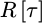values.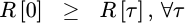Similarly, the autocorrelation function can easily be proven to be conjugate symmetric in time.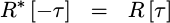This will allow us in a moment to only estimate half of the function, and yet still get the other half for free.

So let’s bring the expectation inside the integral of our PSD expression, and then replace the expectation of `x(t_1)x(t_2)` with this newexpression.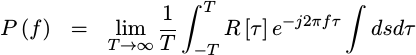The good news is that this function can now easily be integrated across the new value s. The bad news is that the limits have become harder to calculate. For the sake of discussion, I’ll skip this bit of math and just point out that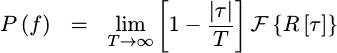as long as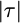is less than `T`.

Now, since this PSD expression no longer depends upon T, we can take our limit and discover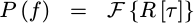This is the conclusion of the Wiener-Khinchin theorem theorem.

What does this mean for the digital designer? Simple. It means that if we estimate, we can later take a Fourier transform of our estimate in order to see what our PSD looks like.

But how to estimate?

## Estimating the AutoCorrelation Function

How shall we go about estimating? Let’s go back to the definition of? and first derive some properties of the autocorrelation function, then it should be easier to see how we might use this,First, since we picked a function that wasn’t dependent upon time, we could just as easily write,The first important property, and one that I’ve mentioned above, is that. I’ll leave this proof for the student. The cool part about this property from a digital designers standpoint is that we only need to estimate one half of. The other half we get for free.

Second, remember how we stated that an expected value could be approximated by an average? Suppose we kept track of some number of prior samples of our signal, defined by however many values of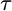we were interested in, and just averaged this product across `N` sample pairs? We might then have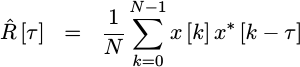If we skip the divide until later, we could rewrite this as,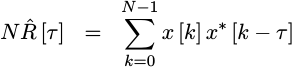which looks very much like a value an FPGA might be able to calculate.

Here’s how this algorithm will work:

1. As samples come in, we’ll store them into an internal buffer–much like we did for the slow filter implementation.

2. Also, for each sample that comes in, we’ll read back an older sample, multiply the two together, and then add the result to a correlation accumulator held in memory indexed by the lag index,.

Note that the first time through, when the number of averages so far is zero, we’d need to write the product to memory rather than adding it to the prior value.

There is another, similar, implementation which we could build that would use a recursive averager here in order to have a result which is always available.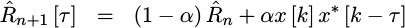Let me invite you to consider building this alternative implementation on your own and then comparing the results. Today, though, we’ll just build a straight block averager.

1. We’ll repeat this for some number of incoming averages, `N`, that we want to calculate.

2. Once we’ve reached the last average, we’ll stop, interrupt the CPU, and wait to be told to start again.

1. The CPU, can then read out the results and restart the spectral estimator to get a new estimate.

Optionally, we might choose to use a double-buffered design, similar to the one we used for the histogram in order to be able to always read out valid results, even when the algorithm is doing its calculations.

That’s the basics of the algorithm. Now, let’s see what it takes to schedule this pipeline.

## Scheduling the Pipeline

As with a lot of algorithms of this type, such as any of our slower filters, we’re going to drive this from a state machine. The state machine will handle when to start, how many averages need to be made, and so forth. The state machine signals will then be used to drive a pipeline of operations for this core. Let’s allow that our state machine creates a signal, `running`, which will be true as long as we continue our averages.

Now let’s work through the signals we might need. We can start with our product. This product will need both our new data, together with the data received by our design some number of clocks ago.

From just this operation alone, we can start working through an initial pipeline schedule.For example, once this operation is complete, we’ll then have a product that we need to add to the prior sum.

The product from our multiply will be available to create this new average on the clock after the multiply, and the new average will be available to be written to memory one clock after that. These values are shown in our initial schedule above, shown in Fig. 3.

A quick look at these signals will quickly reveal that they aren’t nearly sufficient for an algorithm like this one. For example, where does the `delayed_data` signal come from? It must be read from memory. That will require a delayed signal address to be valid one clock earlier. Likewise, the new data will need to be captured one clock earlier–but only at the beginning of our run.

Let’s flesh this schedule out a bit more in Fig. 4 below.Moving one clock forward, in order to have a previous average in the “Product Result” clock, we need an address in the prior clock period. I also chose that prior clock period, “Product Operands”, to be the period where the state variables would be valid, and so I created a `running` state flag to indicate being in the state where I was walking through all of the different lags, calculating a product on each lag.

Now, I can finally read the prior average,

I also need to delay this address by two clocks within the pipeline in order to write the updated average data back, so I created a temporary address value to do this followed by the actual write address for the updated average data.

That’s almost the whole pipeline, save one problem: on our first set of averages, we want to make certain that we overwrite the average data. This requires a flag, I’ll call it `first_round`, indicating that the average data should be just the product of the data with the delayed data–not that plus the prior average.

This `first_round` flag, however, has to come from our state machine which … is valid one round prior. So, one round, prior I created a `clear_memory` flag which would be true any time the number of prior averages, `avcounts`, was zero.

At least, that’s the basic algorithm design.

## Bootstrapping the Simulation

Formally verifying a DSP algorithm can be a real challenge simply for the fundamental reason that most formal verification tools can’t handle multiplies. Still, all I wanted from my design initially was to see a trace that would walk through all the operations of the core and set the interrupt output to indicate it had done everything. Consider how much work that takes to do in simulation,

Yes, I did eventually verify this core using simulation so I have the Verilator C++ code necessary to do it. But let’s now compare that to the amount of code necessary to just create a trace showing this design in operation using SymbiYosys,

Of course, when a `cover()` statement doesn’t produce the result you want, you might find yourself in a bind not knowing what went wrong. So I added a couple extra cover statements to help me know how long I would need to wait for the outgoing interrupt, `o_int`, to be true.

There was one other key component to creating a trace of the logic within this core using SymbiYosys, and that was reducing the number of lags checked and the number of averages until the entire operation would fit into a reasonably sized trace. While every tool has their own way of adjusting parameters at build time, SymbiYosys allows embedded Python scripts to accomplish the task. Hence, the following lines in my SymbiYosys script trimmed this `cover()` check down to something usable,

The entire SymbiYosys script is posted, together with the algorithm on-line, so feel free to examine it and compare with the Verilator simulation code.

As I mentioned above, I also ran simulations on this core (eventually), but we can come back to that in a moment. For now, let’s back up and discuss the state machine signals for a moment based upon the trace SymbiYosys generated and shown below in Fig. 5.The whole operation starts out with a `start_request`, set by either the bus or a reset. This start request clears the count of the number of averages, `avcount`, sets a flag to indicate that we’ll be clearing our average memory, `clear_memory`, and sets another flag to indicate that we wish to be responsive to the next data item that comes in, `check_this`.

Once a new data value comes in, as indicated by `i_data_ce && !running && check_this`, the core starts calculating products and adding them to the running autocorrelation accumulators. Once it has gone through all of the various lags contained in those accumulators, the core then waits for another data item to come in.

What if other data items come in while the core is busy? They are ignored. We can do this because we’ve already assumed that our signal was wide-sense stationary, and so which value we use to run our estimates on shouldn’t matter, right? Well, there is an unfortunate consequence to this. Specifically, if you provide the core with a cyclostationary signal, one whose statistics repeat in time every `1+2^LGLAGS` system clocks, you might not get a representative result. If you think that’s your case, you can include a random number into the start check, so as to randomly spread out which values you check and thus guarantee a more uniform rather than periodic sample set–but that would be a (slightly) more advanced design than the one we are working on today.

Suppose we zoom in on this trace a bit more, and see what’s going on while the core is `running`? There’s a lot that happens there, and it’s worth understanding.First, any time new data comes in, it is written to memory and the `data_write_address` is incremented. If that new data comes in when the autocorrelation updater is already `running`, it simply gets written to memory but otherwise ignored. This is why the `new_data` trace above can appear to skip values, such as jumping from `D4` to `D9`, but also increment from one value to the next, such as from `D9` to `DA` to `DB` when the data arrives slow enough.

If the averager isn’t running, however, this new data sample is taken to run correlations on with past data. It’s important, for pipeline purposes, that the `delayed_address` be one greater than the `data_write_address` on this clock. That helps to guarantee that no matter what data comes in while we are processing, the most recent data will always be read back in time to calculate a zero lag. You can see this in Fig. 6 above by the fact that the `delayed_data` that’s read from memory always equals the new data on the last period while the design is `running`–regardless of how fast new data comes in.

On the clock period after the `running` state machine signal, the address of the prior average for this lag is valid. That gets read into `last_average` on the next clock, and then added to the `product` and written out on the clock after as the `new_average`.

That’s essentially all of the major design details. The cool part is that I was able to see all of these details from the `cover()` trace produced above–at least enough to organize it into the charts you see in both Figs. 5 and 6 above.

Let’s walk through how all this logic fits together into the next section.

## The Cheap Spectral Estimator Design

If you aren’t interested in the gory details of this algorithm, feel free to skip forward a section to see how well the algorithm works. On the other hand, if you want to try building something similar, you might enjoy seeing how the inner details work. Surprisingly, they aren’t all that different from how our prior slow filter worked: there’s one multiply that’s shared across operations and some block RAMs to contain any data we need to keep track of.

Our core’s portlist footprint isn’t really much more than that of any generic Wishbone slave. The biggest difference is that we have the `i_data_ce` signal to indicate new data is valid, and the `i_data` signal containing any new samples from our signal.

We also output an interrupt, `o_int`, any time the core completes its averaging and has an autocorrelation estimate available to be examined.

Next, we’ll need to decide how we want to configure this core.

First, we’ll need to know how many lags we wish to check. That is, how big, or rather how long, should our correlation function estimate be? This is important. The more samples in your estimate, the more freuency “resolution” your PSD will have, but also the longer it will take the result will take to converge.

The parameter of `LGLAGS=6` sets the number of lags calculated to `2^6 = 64`. It’s not amazing, but it will work for most testing and verification purposes.

We’ll also allow our incoming data to have an implementation dependent bit-width.

Finally, we need to know how many averages to do. The more averages you do, the less jumpy the resulting estimate will be and the closer it will hold to the actual autocorrelation. However, the more averages you do, the longer it will take to get an estimate of your signal. You, as the designer, will need to make that tradeoff.

Here, we just specify the number of averages as a log based two number. Fifteen this corresponds to averaging `2^15 = 32,768` points together to get our spectral estimate.

Again, this only works if the incoming signal truly was wide-sense stationary. While very few signals truly are, the approximation is still useful in real life. Just beware that if the environment changes mid-average, then you won’t necessarily see the change in the results.

Two more parameters, the number of address bits for our bus interface and the width of the bus, are just useful for making the design easier to read below.

For your sake, I’ll spare the port and signal definitions that follow, but you are more than welcome to examine the details of the core itself if you would like.

The first block of logic within the core involves writing incoming data to memory. Every new data value will get written to memory regardless of whether or not it drives a summation round below.

Note that there’s no reference to the reset signal here. This was a careful design choice made on purpose. First, you can’t reset block RAM. Sorry, it just doesn’t work. Second, any data flow will (eventually) work its way through whatever junk was in the data registers initially. Finally, this logic keeps our last read data values always valid.

Where it gets annoying is when we try to build a simulation with a known input and a (hopefully) known output, but that’s another story.

The next piece is the start request that starts off our whole estimate. This core will immediately estimate the autocorrelation of an incoming waveform immediately on startup, on reset, or upon any write to the core.

Once the core has started running in response to this start request, the request line will clear itself.

The `check_this` signal below is used to indicate we want to calculate an autocorrelation estimate. It gets set on any start request, but also automatically clears itself after the last average. In this fashion, we can hold onto an average until it is read before using it again.

Once we’re all done, we’ll want to set an interrupt wire for the CPU to examine. That’s the purpose of `o_int` below. It will be set during the last average set once the last value is written to the average memory.

The next item in our state machine is the counter of the number of averages we’ve calculated. We’ll clear this on any start request, and otherwise increment it at the end of any processing group–as shown in Fig. 5 above.

As shown in our pipeline schedules and figures above, its important to calculate the address for the prior signal data, `delayed_addr`, so that it’s valid when `!running`. Further, it needs to be valid on all but the last clock of `running`. Finally, the value is critical. When the data that we are going to examine gets written, that is when `i_data_ce && !running`, this address must already be equal to the next data memory address following the write address. That helps to 1) give us immunity to the data speed, while also 2) guaranteeing that the last value we examine is the value just received and so contributes to the `R` average.

The extra variable here, `last_read_address`, is used as an indication that we need to base the next value on the current address of the next data that will come in, `data_write_address`, rather than just continuing to walk through older data.

The address for the reading from the average accumulator memory is called `av_read_addr`. We always walk through this address on every run from start, `av_read_addr==0`, to stop, `&av_read_addr`. When not running, we go back to the first address to prepare for the next round.

If you look through the trace in Fig. 6 above, there’s really an extra clock in there over and above what needs to be there. This is one of those pieces of logic that would need to be updated to get rid of that extra clock.

Personally, I’m okay with the extra clock if for no other reason than the number of clock cycles required for any averaging cycle, `1+2^LGLAGS`, tends to be relatively prime to most digital artifacts and so (perhaps) keeping it this size will help reduce any non-WSS artifacts.

That really only leaves two state machine signals left to define, the `running` and `clear_memory` flags. As you may recall from our pipeline schedule, `running` will be true while we are working through the various products of our autocorrelation estimate. It gets set whenever new data comes in that we are expecting, i.e. whenever `i_data_ce && check_this` value. It gets cleared once we read from the last address of our autocorrelation average memory.

The `clear_memory` flag has a very similar set of logic. This is the flag that’s used to determine if we want to rewrite our accumulators versus just adding more values to them. For the first averaging pass, we’ll want this value to be set–so we set it on any reset or following any `start_request`. We then clear it at the same time we clear the `running` flag.

Finally, in order to know when to write to our memory, we’ll want to delay the `running` indicator through our pipeline. That’s the purpose of the `run_pipe` signal below.

It’s now time to walk through the various stages of the pipeline processing.

If you examine the code, you’ll see I’ve placed a comment block at the beginning of the logic for each pipeline stage, to help me keep track of what’s being done when. Indeed, this was how I originally scheduled the pipeline in the first place–I only drew Fig. 3 after the entire design was up and running.

After getting the various signals associated with each clock wrong a couple of times, I started adding to the comment block the list of signals defined by each block. When that wasn’t enough, I then added to the block the signal(s) that defined when the pipeline stage was active. In this case, clock 0 takes place one clock before the `running` clock, as shown in Fig. 4 above in the cycle I called “Incoming data”.

It’s on this clock that we need to copy the incoming data to our `new_data` register, to be used in our subsequent autocorrelation calculations. This helps to make certain that it doesn’t change on us while running.

Similarly, we’ll want to read the delayed data from memory.

It’s on the next clock that we’ll multiply these two values together.

The biggest and most important part of this next clock cycle is multiplying our two data values together.

Notice how I have two versions of this multiply above. The first version, used when we are using formal verification, allows the multiplication result to be (roughly) anything. (I constrain it more in the formal section that follows.) The second version is how it would work normally.

The `clear_memory` flag is true on this clock, but we won’t add this product together with the lag product for another clock. On that clock we need to know if it’s the first round or not, so let’s move this value one clock further into our pipeline.

The last thing we need from this clock cycle is the previous value from the accumulator memory. That’s as easy as just reading it.

Unlike the histogram design, we don’t need to worry about operand forwarding during this calculation. When we read this value, we can rest assured that it won’t also be present in any of the two subsequent stages. That will nicely simplify the logic that follows.

Moving on to the next clock, we now want to add our product and last average together.

I’ve chosen to be explicit with the sign bit extensions here to avoid Verilator warnings, but this should be equivalent to `last_average + product`.

I also need to move the accumulator read address from the last pipeline stage into the next one. This can be done with a simple shift register, although I’ll admit I did get the length of this register wrong once or twice. This is now the correct width with `av_tmp_addr` having the same number of bits as `av_write_addr`.

The last task in this pipeline stage is to determine if this is the last write block. If it is, we can set the interrupt above. It would be a bit of a problem, however, for the CPU to restart this core only to get an interrupt from a prior calculation. To suppress this extra interrupt, we reset this flag on either a system reset, `i_reset`, or a user restart of the core.

That brings us to the third clock.

During this clock, all we need to do is write the data back to memory.

When should we write? Two clocks after `running`–which is what `run_pipe` keeps track of for us.

The last piece of this core is handling bus interactions.

If you are using Wishbone, this is really easy. Indeed, it’s as easy as the simple Wishbone slave we discussed some time ago.

We hold the stall line low,

and acknowledge any bus request one clock later.

I know the original simple Wishbone slave didn’t have the check on `i_reset`, but I’ve learned a bit since using formal methods. Two particular things formal methods have taught me are first to use the initial value, and second to reset the acknowledgment.

The last step would be to read data from the averages register. Ideally, this should look like,

Except … that’s not good enough. In particular, the average memory, `avmem`, has a width given by `2*IW+LGNAVG` which might or might not match the bus width of `o_wb_data.

So I’m going to read into a register of exactly the right length first,

and I’ll expand into the right width in the next step.

If the two widths match, I can just keep `o_wb_data` the same as this `data_out` value.

At the next step, though, I need to admit I broke a working design by not doing this right. Obviously, if there aren’t enough bits, you’d want to sign extend to the right number of bits.

Here’s the problem I ran into: I was running simulation tests with `2^1024` lags and `10` bit data, and `1024` averages. When simulations took too long and the data didn’t quite look right (it was close), I adjusted the design to examine `2^64` lags and `32768` averages. All of a sudden my correlation results were all broken.

As an example, if you give the core a sine wave input, you should be able to read a cosine autocorrelation output, as shown in Fig. 7.However, when I looked at the autocorrelation of a sinewave, I received the autocorrelation output shown in Fig. 8 below.The first indication that something is broken is the fact that `R` isn’t a maximum. The second indication, the fact that the measured waveform looks nothing like what it should.

It took me quite a bit of time to find this bug. It got so far that I was chasing it in a simulation waveform, and the autocorrelations all looked right. Then I noticed the number of bits in `data_out`–35. The top three bits were getting dropped in the assignment above (which wasn’t originally contingent on the number of bits).

A sadder man but wiser now, I’ve learned to check for all possible differences between the number of bits in `data_out` and the bus width. The check above, and the third case (below) fixes this issue.

That’s the logic necessary to build this core.

But does it work?

## Simulation Results

If you look over the core, you’ll find a formal proof that the `R` value is positive, and that the accumulator hasn’t overflowed. While that’s nice, it doesn’t really compare the core’s performance against a known input to know that it produces a known output. To handle that, I tried seven known inputs using a Verilator simulation script.

1. Random Noise

The classic autocorrelations test is random noise. If noise values have a known variance,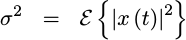and if noise values are equally likely positive as they are negative, then it’s not all that hard to prove that the correct correlation would be given by,Of course, this is only the true autocorrelation, depending upon the mathematical expectation operator, which itself is dependent upon a fictitious probability density function which may or may not match reality. Unfortunately, the algorithm above only estimates the autocorrelation and the estimate may or may not equal the expected value. Sure, over time it will get closer, but how shall I easily tell if the current value is close enough to declare that my simulated algorithm “passes” its simulation check?Having the right shape above is at least a good start, so let’s keep going.

1. All zeros

After my frustrations with the random data, I thought to try some carefully chosen known inputs for testing. The easiest of these to work with was the all zeros incoming sequence. Clearly if the inputs are all zero, then the autocorrelations estimate should be zero as well.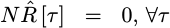Unfortunately, the all zeros sequence doesn’t really create anything pretty to plot and display. Hence, while the test (now) works, it doesn’t leave much to show for it.

1. All ones

The problem with the all zeros sequence is just the large number of broken algorithms that would still pass such a test. Therefore I thought I’d create a third test where the inputs were all ones. As with the all zeros test, the result should be absolutely known when done.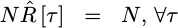Sadly, as with the all zeros test, the result isn’t very interesting and so not really worth plotting.

1. Alternating ones and negative ones

What if we alternate plus and minus ones on the input? Again, the estimate of the autocorrelations should match the actual autocorrelations, withNow we can finally plot something of marginal interest. In this case, we can also compare the expected autocorrelation against the measured one.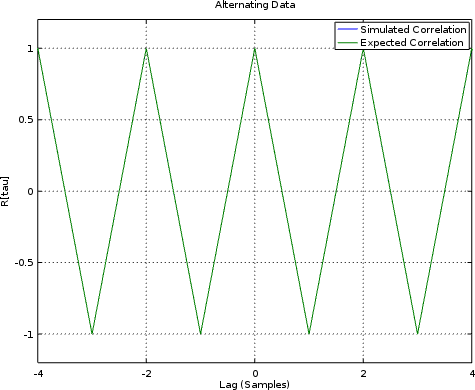The two are clearly shown on top of each other, so we can mark this test down as a success.

1. Alternating ones and negative ones, only slower

I then tried to create a similar signal that would alternate ones and negative ones, just slower: seven `1`s followed by seven `-1`s in a repeating sequence. The only problem was, I didn’t really work out the math to know what the “right” answer was or should be. As a result, although I can plot the result, it’s harder to tell whether it works or not.

2. A Sinewave

That leads to another autocorrelation test that might be of interest: what would happen if we gave this algorithm a sinewave as an input?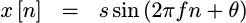This is another one of those “classic” inputs, where the result should match a known pattern. Indeed, with just a little math, it’s not hard to prove what the autocorrelation result should be.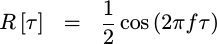Unfortunately, as with the first test case, the core will only produce an estimate of the true autocorrelation function, rather than an exact autocorrelation result. Worse, with a little work, you’ll realize that multiplying two sine function values together will result in a cosine plus another trigonometric term. The second term should average out, but I haven’t yet tracked out what it’s limits are and whether or not the average is causing this second term to average out as fast as it should.

Still, if you compare the sinewave spectra with the expected spectra in Fig. 11 below, the two land nicely on top of each other.That suggests that we’re at least on the right track.

I will admit that when I looked at this shape for the first time I was a little confused. The “correct” spectra for a sinewave should be a single peak, right?

Sadly, no. The “correct” spectra of a sinewave should be two peaks–one at the positive sinewave frequency and one at the negative frequency. (I was using complex FFTs.) Not only that, but the sinewave’s spectra should consist of two sinc functions superimposed upon each other, and so this result does look right–even if it wasn’t what I was expecting at first.

1. A Random Binary Waveform

Let’s finally move on to a last test case, and pick something more likely to be a useful demonstration. So, let’s pick a random binary waveform. This waveform will contain a series of bit-periods where the input to the core will be either plus or minus full scale, `+/- (2^{IW-1}-1)`.

As with the sine wave, the true autocorrelation of such a waveform has a closed form representation,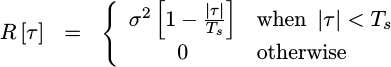where `sigma^2` is the absolute value of the signal.

Much to my chagrin, I only got close to this estimate, as shown in Fig. 12.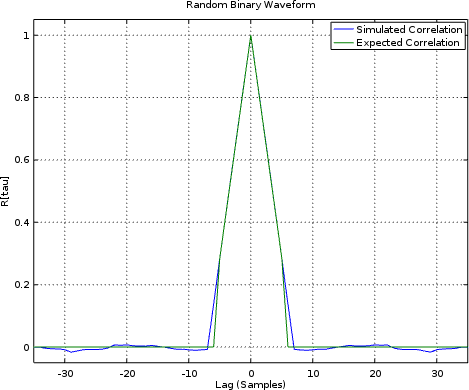Was it close enough? Perhaps, but what I’d really like to do is to display the PSD of such a signal, as shown in Fig. 13 below.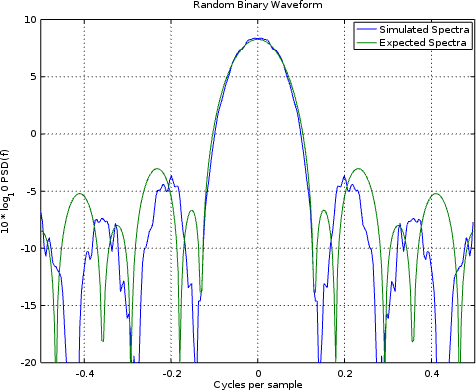In this case, we got close again. Unfortunately, it wasn’t close enough to match the expected shape over 10dB down.

Still, all told, we’ve done a decent job of estimating the PSD of a signal within our FPGA, but without actually running any FFTs on the FPGA to do it.

## Conclusion

If your goal is to grab a picture of what the spectral content is of a signal within your design, but without using a lot of logic, then I’d say we’ve achieved it with this little design. No, it’s not perfect, and yes there’s some amount of variability in the estimated waveforms–this is all to be expected. The cool part is that this technique only uses one multiply and a couple of block RAM memories.

Will this fit on an iCE40 UP5k, together with other things? Indeed, there’s a good chance I’ll be able to add this in to some of my SDR designs to “see” what’s coming in from the antenna. Indeed, given the speed and simplicity of this algorithm, I might even be able to handle the incoming data at the 36MHz data rate! There’s no way I could’ve done that with an FFT approach–not on this hardware at least.